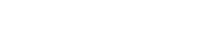# ISA Certified Control Systems Technician (CCST) program

• Certification Review

Certified Control System Technicians (CCSTs) calibrate, document, troubleshoot, and repair/replace instrumentation for systems that measure and control level, temperature, pressure, flow, and other process variables.

### CCST question

A 12-volt battery rated to produce 2 amps of current for 30 hours is connected to a 400 ohm load. How long should the battery remain an energy producing capability?

A. 2 hours
B. 100 hours
C. 1500 hours
D. 2000 hours

The correct answer is D: "2000 hours".

The capacity of the battery can be determined by the current availability multiplied by the length of time that the battery can output that current.  In this case,

2 amps x 30 hours = 60Amp-hours, or 60Ah

When a load is connected to the battery, the amount of continuous current draw can be determined by Ohm's Law:

E = I x R

I = E / R

I = 12 / 400 = 0.03A

Then, the time it will take for the battery to discharge under these conditions (0.03A continuous current draw) is given by:

Discharge time (hours) m = 60 Amp-hours / 0.03 A

= 2000 hours

Reference: Goettsche, L.D. (Editor), Maintenance of Instruments and Systems, 2nd Edition (2005), ISA Press